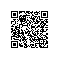# JavaScript实现集合与字典

JavaScript实现集合与字典

1.1.简介

JavaScript中的Object类中的key就是一个集合，可以使用它来封装集合类Set。

remove（value）：从集合中移除一个值；
has（value）：如果值在集合中，返回true，否则返回false；
clear（）：移除集合中的所有项；
size（）：返回集合所包含元素的数量，与数组的length属性相似；
values（）：返回一个包含集合中所有值的数组；

1.2.代码实现

//封装集合类
function Set() {
//属性
this.items = {}

//方法
//一.has方法
Set.prototype.has = value => {
return this.items.hasOwnProperty(value)
}

//判断集合中是否已经包含该元素
if (this.has(value)) {
return false
}
//将元素添加到集合中
this.items[value] = value//表示该属性键和值都为value
return true//表示添加成功
}

//三.remove方法
Set.prototype.remove = (value) => {
//1.判断集合中是否包含该元素
if (!this.has(value)) {
return false
}

//2.将元素从属性中删除
delete this.items[value]
return true
}

//四.clear方法
Set.prototype.clear = () => {
//原来的对象没有引用指向，会被自动回收
this.items = {}
}

//五.size方法
Set.prototype.size = () => {
return Object.keys(this.items).length
}

//获取集合中所有的值
//六.values方法
Set.prototype.values = function() {
return Object.keys(this.items)
}
}

//测试集合类
//1.创建Set类对象
let set = new Set()

//添加元素

//3.测试values方法
console.log(set.values());                                      //74

//删除元素
//4.测试remove方法
console.log(set.remove('a'));                                   //78
console.log(set.remove('a'));                                   //79
console.log(set.values());                                      //80

//5.测试has方法
console.log(set.has('b'));                                      //83

//6.测试size方法和clear方法
console.log(set.size());                                        //86
set.clear()
// 由于clear方法的实现原理为指向另外一个空对象，所以不影响原来的对象
console.log(set.size());                                        //89
console.log(set.values());                                      //90

1.3.集合间的操作

Set.prototype.union = otherSet => {

  // this:集合对象A
// otherSet:集合对象B
//1.创建一个新的集合
let unionSet = new Set()

//2.将A集合中的所有元素添加到新集合中
let values = this.values()
// for(let i of values){
// }
for(let i = 0;i < values.length;i++){
}

//3.取出B集合中的元素,判断是否需要加到新集合中
values = otherSet.values()
// for(let i of values){
// }
for(let i = 0;i < values.length;i++){
}
return unionSet
}

Set.prototype.intersection = otherSet => {

  // this:集合A
// otherSet:集合B
//1.创建新的集合
let intersectionSet = new Set()

//2.从A中取出一个元素，判断是否同时存在于集合B中，是则放入新集合中
let values = this.values()
for(let i =0 ; i < values.length; i++){
let item = values[i]
if (otherSet.has(item)) {
}
}
return intersectionSet
}

Set.prototype.diffrence = otherSet => {

    //this:集合A
//otherSet:集合B
//1.创建新的集合
var diffrenceSet = new Set()

//2.取出A集合中的每一个元素，判断是否同时存在于B中，不存在则添加到新集合中
var values = this.values()
for(var i = 0;i < values.length; i++){
var item = values[i]
if (!otherSet.has(item)) {
}
}
return diffrenceSet
}

Set.prototype.subset = otherSet => {

    //this:集合A
//otherSet：集合B
//遍历集合A中的所有元素，如果发现，集合A中的元素，在集合B中不存在，那么放回false，如果遍历完整个集合A没有返回false，就返回true
let values = this.values()
for(let i = 0; i < values.length; i++){
let item = values[i]
if(!otherSet.has(item)){
return false
}
}
return true
}

2.1.简介

set(key,value)：向字典中添加新元素。
remove(key)：通过使用键值来从字典中移除键值对应的数据值。
has(key)：如果某个键值存在于这个字典中，则返回true，反之则返回false。
get(key)：通过键值查找特定的数值并返回。
clear()：将这个字典中的所有元素全部删除。
size()：返回字典所包含元素的数量。与数组的length属性类似。
keys()：将字典所包含的所有键名以数组形式返回。
values()：将字典所包含的所有数值以数组形式返回。
2.2.封装字典

//封装字典类
function Dictionary(){
//字典属性
this.items = {}

//字典操作方法
//一.在字典中添加键值对
Dictionary.prototype.set = function(key, value){

this.items[key] = value

}

//二.判断字典中是否有某个key
Dictionary.prototype.has = function(key){

return this.items.hasOwnProperty(key)

}

//三.从字典中移除元素
Dictionary.prototype.remove = function(key){

//1.判断字典中是否有这个key
if(!this.has(key)) return false

//2.从字典中删除key
delete this.items[key]
return true

}

//四.根据key获取value
Dictionary.prototype.get = function(key){

return this.has(key) ? this.items[key] : undefined

}

//五.获取所有keys
Dictionary.prototype.keys = function(){

return Object.keys(this.items)

}

//六.size方法
Dictionary.prototype.keys = function(){

return this.keys().length

}

//七.clear方法
Dictionary.prototype.clear = function(){

this.items = {}使用钉钉扫一扫加入圈子
+ 订阅